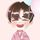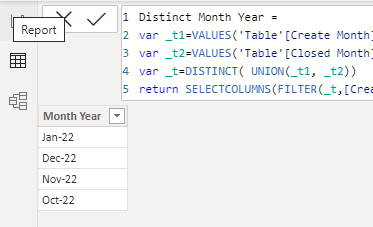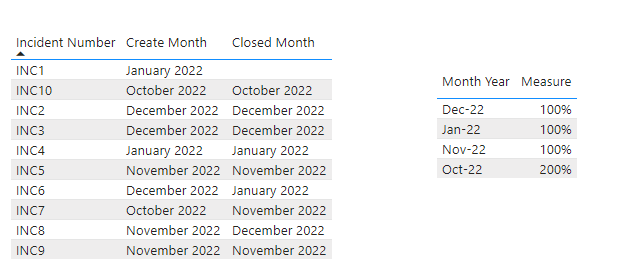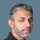cancel
Showing results for
Did you mean:Frequent Visitor

## Ratio of Closed Tickets in Month

Hi,

Can someone please help me in calculating a measure on ratio of Closed Incidents in a month.

Criteria is for any given month, find ratio of closed incidents compared to open incidents. As an example, in the below table, if I want to calculate the ratio for month of Jan-22, it should be 100% (i.e. 2 x closed in Jan / 2 x opened in Jan).

 Incident Number Create Month Closed Month INC1 Jan-22 INC2 Dec-21 Dec-21 INC3 Dec-21 Dec-21 INC4 Jan-22 Jan-22 INC5 Nov-21 Nov-21 INC6 Dec-21 Jan-22 INC7 Oct-21 Nov-21 INC8 Nov-21 Dec-21 INC9 Nov-21 Nov-21 INC10 Oct-21 Oct-21

Thanks

1 ACCEPTED SOLUTIONCommunity Support

Hi @gauravg3 ,

Since there are two date columns with different values, I suggest you create a new table with all distinct Month-Year:

``````Distinct Month Year =
var _t1=VALUES('Table'[Create Month])
var _t2=VALUES('Table'[Closed Month])
var _t=DISTINCT( UNION(_t1, _t2))
return SELECTCOLUMNS(FILTER(_t,[Create Month]<>BLANK()),"Month Year",FORMAT([Create Month],"mmm-yy"))``````And then calculate the %:

``````Measure =
var _create=CALCULATE(COUNTROWS('Table'),FILTER('Table',FORMAT([Create Month],"mmm-yy")=MAX('Distinct Month Year'[Month Year])))
var _close=CALCULATE(COUNTROWS('Table'),FILTER('Table',FORMAT([Closed Month],"mmm-yy")=MAX('Distinct Month Year'[Month Year])))
return DIVIDE(_create,_close)``````

Output:Best Regards,
Eyelyn Qin
If this post helps, then please consider Accept it as the solution to help the other members find it more quickly.

5 REPLIES 5Community Support

Hi @gauravg3 ,

Since there are two date columns with different values, I suggest you create a new table with all distinct Month-Year:

``````Distinct Month Year =
var _t1=VALUES('Table'[Create Month])
var _t2=VALUES('Table'[Closed Month])
var _t=DISTINCT( UNION(_t1, _t2))
return SELECTCOLUMNS(FILTER(_t,[Create Month]<>BLANK()),"Month Year",FORMAT([Create Month],"mmm-yy"))``````And then calculate the %:

``````Measure =
var _create=CALCULATE(COUNTROWS('Table'),FILTER('Table',FORMAT([Create Month],"mmm-yy")=MAX('Distinct Month Year'[Month Year])))
var _close=CALCULATE(COUNTROWS('Table'),FILTER('Table',FORMAT([Closed Month],"mmm-yy")=MAX('Distinct Month Year'[Month Year])))
return DIVIDE(_create,_close)``````

Output:Best Regards,
Eyelyn Qin
If this post helps, then please consider Accept it as the solution to help the other members find it more quickly.Super User

@gauravg3

Before answering your question, I would like to know if you have a dates table in your model and how the model is organized, this will help provide a better answer. share screenshot of your model

Did I answer your question? Mark my post as a solution! and hit thumbs upFrequent Visitor

There is no separate date table in the model...All the values are in the same table.Super User

@gauravg3 , Please refer to the blog on the same topic. The open ticket is same as a current employee, Hire is this month new, terminate is closed

https://community.powerbi.com/t5/Community-Blog/HR-Analytics-Active-Employee-Hire-and-Termination-tr...Frequent Visitor

Hi Amit,

I tried the calculations mentioned in your separate post, but the output is same for all months on each calculation. Is there any other way to get % of closed tickets for each month.